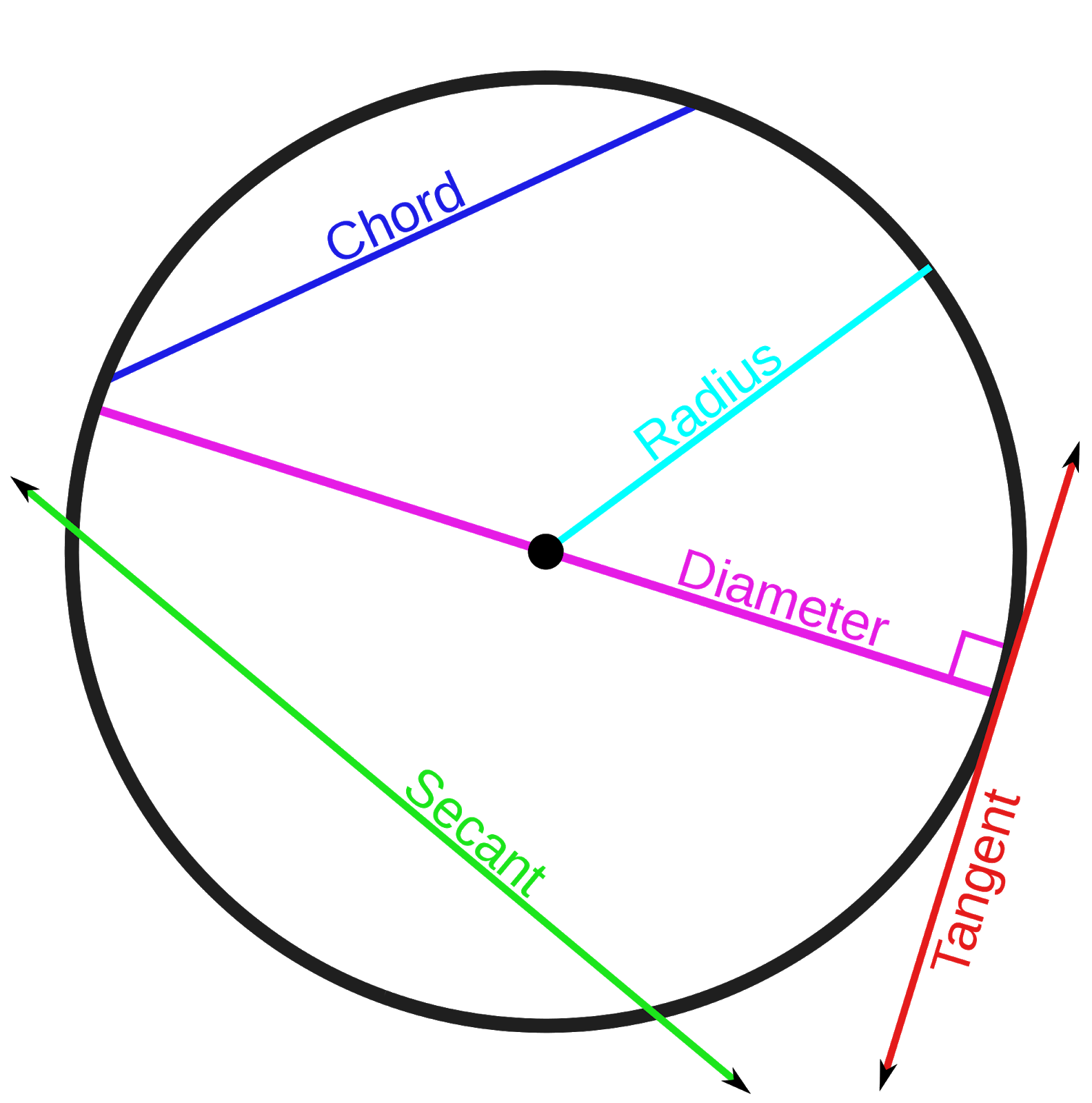# Circles

## Parts of a circle

You will need to make sure you can identify all aspects of a circle.

Let’s start with some key lines that make elements of a circle.The radius is always half the diameter.

The diameter goes across the circle, through the middle of the circle.

A chord it is a line that touches the circumference at two points but does not cross the middle of the circle.

A __tangent __ to the circle touches the circle at only one point.

The __circumference __of the circle (it’s like the perimeter of the circle).

A secant is a line that intersects the circle in two different points.An arc this is part of the circumference.

A sector it is part of the circle enclosed by two radius or by two points on the circumference.

An area not enclosed by two radii is called a segment.

What is the name for the area of a circle between the circumference and two radii?
Sector
What is half the diameter?
Radius
What touches a circle at one point?
Tangent